﻿ 高精度Fox-Goodwin时间积分法

# 高精度Fox-Goodwin时间积分法Highly Accurate Fox-Goodwin Time Integration Method

Abstract: A Highly Accurate Fox-Goodwin time Integration Method (HAFIM) is presented in this paper. HAFIM creates the highly accurate Jacobi matrix by multi-sub-step notion and uses the highly ac-curate Jacobi matrix for recursive procedure. In the process of constructing the matrix, the exponential matrix operation techniques are employed to reduce the computational efforts and the computer rounding error. The analysis on properties indicates that the proposed method possesses higher amplitude and phase accuracy compared with the Precise time Integration Methods (PIMs). Moreover, the proposed method is very practical given its simplicity.

1. 引言

2. 算法格式

2.1. 动力学方程

$M\stackrel{¨}{x}+C\stackrel{˙}{x}+Kx=R\left(t\right)$ (1)

$R\left(t\right)=Tf\left(t\right),f\in \left\{f\left(t\right)|\stackrel{¨}{f}+B\stackrel{˙}{f}+Df=0,B,D为常矩阵\right\}$ (2)

$\left[\begin{array}{cc}M& O\\ O& I\end{array}\right]\left[\begin{array}{c}\stackrel{¨}{x}\\ \stackrel{¨}{f}\end{array}\right]+\left[\begin{array}{cc}C& O\\ O& B\end{array}\right]\left[\begin{array}{c}\stackrel{˙}{x}\\ \stackrel{˙}{f}\end{array}\right]+\left[\begin{array}{cc}K& -T\\ O& D\end{array}\right]\left[\begin{array}{c}x\\ f\end{array}\right]=\left[\begin{array}{c}0\\ 0\end{array}\right]$ (3)

$R\left(t\right)\cong {a}_{0}+\underset{n=1}{\overset{q}{\sum }}\left({a}_{n}\mathrm{cos}\left(\frac{2n\text{π}t}{{T}_{0}}\right)+{b}_{n}\mathrm{cos}\left(\frac{2n\text{π}t}{{T}_{0}}\right)\right)$ (4)

$\begin{array}{l}T=\left[\begin{array}{ccccccc}{a}_{0}& {a}_{1}& \cdots & {a}_{q}& {b}_{1}& \cdots & {b}_{q}\end{array}\right]\\ f={\left[\begin{array}{ccccccc}1& \mathrm{sin}\left(\frac{\text{π}t}{{T}_{0}}\right)& \cdots & \mathrm{sin}\left(\frac{q\text{π}t}{{T}_{0}}\right)& \mathrm{cos}\left(\frac{\text{π}t}{{T}_{0}}\right)& \cdots & \mathrm{cos}\left(\frac{q\text{π}t}{{T}_{0}}\right)\end{array}\right]}^{\text{T}}\\ B=O\\ D=\left[\begin{array}{ccccccc}0& 0& \cdots & 0& 0& \cdots & 0\\ 0& {\left(\frac{\text{π}t}{{T}_{0}}\right)}^{2}& \cdots & 0& 0& \cdots & 0\\ ⋮& ⋮& \ddots & ⋮& ⋮& \ddots & ⋮\\ 0& 0& \cdots & {\left(\frac{q\text{π}t}{{T}_{0}}\right)}^{2}& 0& \cdots & 0\\ 0& 0& \cdots & 0& {\left(\frac{\text{π}t}{{T}_{0}}\right)}^{2}& \cdots & 0\\ ⋮& ⋮& \ddots & ⋮& ⋮& \ddots & ⋮\\ 0& 0& \cdots & 0& 0& \cdots & {\left(\frac{q\text{π}t}{{T}_{0}}\right)}^{2}\end{array}\right]\end{array}$ (5)

$\stackrel{˜}{M}\stackrel{¨}{\stackrel{˜}{x}}+\stackrel{˜}{C}\stackrel{˙}{\stackrel{˜}{x}}+\stackrel{˜}{K}\stackrel{˜}{x}=0$ (6)

$\stackrel{˜}{x}=\left[\begin{array}{c}x\\ f\end{array}\right],\stackrel{˜}{M}=\left[\begin{array}{cc}M& O\\ O& I\end{array}\right],\stackrel{˜}{C}=\left[\begin{array}{cc}C& O\\ O& B\end{array}\right],\stackrel{˜}{K}=\left[\begin{array}{cc}K& -T\\ O& D\end{array}\right]$ (7)

2.2. 求解格式

${z}_{k+1}=A\left(h\right){z}_{k}$ (8)

$\begin{array}{l}\stackrel{˜}{M}{\stackrel{¨}{\stackrel{˜}{x}}}_{k+1}+\stackrel{˜}{C}{\stackrel{˙}{\stackrel{˜}{x}}}_{k+1}+\stackrel{˜}{K}{\stackrel{˜}{x}}_{k+1}=0\\ {\stackrel{˜}{x}}_{k+1}={\stackrel{˜}{x}}_{k}+h{\stackrel{˙}{\stackrel{˜}{x}}}_{k}+{h}^{2}\left(\frac{5}{12}{\stackrel{¨}{\stackrel{˜}{x}}}_{k}+\frac{1}{12}{\stackrel{¨}{\stackrel{˜}{x}}}_{k+1}\right)\\ {\stackrel{˙}{\stackrel{˜}{x}}}_{k+1}={\stackrel{˙}{\stackrel{˜}{x}}}_{k}+h\left(\frac{1}{2}{\stackrel{¨}{\stackrel{˜}{x}}}_{k}+\frac{1}{2}{\stackrel{¨}{\stackrel{˜}{x}}}_{k+1}\right)\end{array}$ (9)

$\begin{array}{l}A\left(h\right)=\left[\begin{array}{cc}I-\frac{{h}^{2}}{2}{A}_{1}+\frac{{h}^{3}}{12}{A}_{3}\left({A}_{2}{A}_{1}+\frac{h}{2}{A}_{1}^{2}\right)& hI-\frac{{h}^{2}}{2}{A}_{2}-\frac{{h}^{3}}{12}{A}_{3}\left({A}_{1}-{A}_{2}^{2}-\frac{h}{2}{A}_{1}{A}_{2}\right)\\ -h{A}_{1}+\frac{{h}^{2}}{2}{A}_{3}\left({A}_{2}{A}_{1}+\frac{h}{2}{A}_{1}^{2}\right)& I-h{A}_{2}-\frac{{h}^{2}}{2}{A}_{3}\left({A}_{1}-{A}_{2}^{2}-\frac{h}{2}{A}_{1}{A}_{2}\right)\end{array}\right]\\ {z}_{k}={\left[\begin{array}{cc}{\stackrel{˜}{x}}_{k}^{\text{T}}& {\stackrel{˙}{\stackrel{˜}{x}}}_{k}^{\text{T}}\end{array}\right]}^{\text{T}},\text{}{A}_{1}={\stackrel{˜}{M}}^{-1}\stackrel{˜}{K},\text{}{A}_{2}={\stackrel{˜}{M}}^{-1}\stackrel{˜}{C},\text{}{A}_{3}={\left(\stackrel{˜}{M}+\frac{h}{2}\stackrel{˜}{C}+\frac{{h}^{2}}{12}\stackrel{˜}{K}\right)}^{-1}\stackrel{˜}{M}\end{array}$ (10)

HAFIM用多分步的思想，将步长h等分为N份，在每个分步内使用时间积分法，则其递推格式可写为

${z}_{k+1}={A}_{\text{H}}\left(h\right){z}_{k}$ (11)

${A}_{H}\left(h\right)=\underset{N}{\underset{︸}{A\left({h}_{N}\right)\cdots A\left({h}_{N}\right)}}={A}^{N}\left({h}_{N}\right)$ (12)

1) 2m算法

$N={\text{2}}^{m}$ ，如 $m=\text{2}0$$N=1048576$ ，则仅需m次迭代就可得到AH，相当于执行下列语句

$\text{for}\left(i=0;i (13)

2) 增量算法

$A\left({h}_{N}\right)=I+S\left({h}_{N}\right)$ (14)

$\begin{array}{l}S\left({h}_{N}\right)={h}_{N}\left[\begin{array}{cc}-\frac{{h}_{N}}{2}{A}_{1}+\frac{{h}_{N}^{2}}{12}{A}_{3}\left({A}_{2}{A}_{1}+\frac{{h}_{N}}{2}{A}_{1}^{2}\right)& I-\frac{{h}_{N}}{2}{A}_{2}-\frac{{h}_{N}^{2}}{12}{A}_{3}\left({A}_{1}-{A}_{2}^{2}-\frac{{h}_{N}}{2}{A}_{1}{A}_{2}\right)\\ -{A}_{1}+\frac{{h}_{N}}{2}{A}_{3}\left({A}_{2}{A}_{1}+\frac{{h}_{N}}{2}{A}_{1}^{2}\right)& -{A}_{2}-\frac{{h}_{N}}{2}{A}_{3}\left({A}_{1}-{A}_{2}^{2}-\frac{{h}_{N}}{2}{A}_{1}{A}_{2}\right)\end{array}\right]\\ {A}_{1}={\stackrel{˜}{M}}^{-1}\stackrel{˜}{K},\text{}{A}_{2}={\stackrel{˜}{M}}^{-1}\stackrel{˜}{C},\text{}{A}_{3}={\left(\stackrel{˜}{M}+\frac{{h}_{N}}{2}\stackrel{˜}{C}+\frac{{h}_{N}^{2}}{12}\stackrel{˜}{K}\right)}^{-1}\stackrel{˜}{M}\end{array}$ (15)

$\left(I+S\left({h}_{N}\right)\right)×\left(I+S\left({h}_{N}\right)\right)=I+2S\left({h}_{N}\right)+S\left({h}_{N}\right)×S\left({h}_{N}\right)$ (16)

$\text{for}\text{\hspace{0.17em}}\left(i=0;i (17)

${A}_{\text{H}}\left(h\right)=I+S\left({h}_{N}\right)$ (18)Table 1. Solution procedures of the highly accurate Fox-Goodwin time integration method

3. 算法性能

$\stackrel{¨}{x}+2\xi \omega +{\omega }^{2}x=0$ (19)

3.1. 稳定性

${A}_{\text{F}}=\frac{1}{{\tau }^{2}+12\xi \tau +12}\left[\begin{array}{cc}-4\xi {\tau }^{3}-5{\tau }^{2}+12\xi \tau +12& -8{\xi }^{2}{\tau }^{2}+12\\ 2{\tau }^{4}-12{\tau }^{2}& 4\xi {\tau }^{3}-5{\tau }^{2}-12\xi \tau +12\end{array}\right],\text{\hspace{0.17em}}{z}_{k}={\left[\begin{array}{cc}{x}_{k}& h{\stackrel{˙}{x}}_{k}\end{array}\right]}^{\text{T}}$ (20)

${A}_{\text{T}}=\frac{1}{{\tau }^{2}+4\xi \tau +4}\left[\begin{array}{cc}-{\tau }^{2}+4\xi \tau +4& 4\\ -4{\tau }^{2}& -{\tau }^{2}-4\xi \tau +4\end{array}\right],{z}_{k}={\left[\begin{array}{cc}{x}_{k}& h{\stackrel{˙}{x}}_{k}\end{array}\right]}^{\text{T}}$ (21)

${A}_{\text{C}}=\frac{1}{4\xi \tau +4}\left[\begin{array}{cc}-2\xi {\tau }^{3}-2{\tau }^{2}+4\xi \tau +4& -4{\xi }^{2}{\tau }^{2}+4\\ {\tau }^{4}-4{\tau }^{2}& 2\xi {\tau }^{3}-2{\tau }^{2}-4\xi \tau +4\end{array}\right],{z}_{k}={\left[\begin{array}{cc}{x}_{k}& h{\stackrel{˙}{x}}_{k}\end{array}\right]}^{\text{T}}$ (22)

1) L = 3

${A}_{3}=I+hH+\frac{{h}^{2}}{2}{H}^{2}+\frac{{h}^{3}}{6}{H}^{3}$ (23)

2) L = 4

${A}_{4}=I+hH+\frac{{h}^{2}}{2}{H}^{2}+\frac{{h}^{3}}{6}{H}^{3}+\frac{{h}^{4}}{24}{H}^{4}$ (24)

$H=\left[\begin{array}{cc}-\xi \omega & 1\\ {\xi }^{2}{\omega }^{2}-{\omega }^{2}& -\xi \omega \end{array}\right],{z}_{k}={\left[\begin{array}{cc}{x}_{k}& {\stackrel{˙}{x}}_{k}+\xi \omega {x}_{k}\end{array}\right]}^{\text{T}}$ (25)

3.2. 精度

$x\left(t\right)={\text{e}}^{-\xi \omega t}\left({c}_{1}\mathrm{cos}{\omega }_{\text{d}}t+{c}_{2}\mathrm{sin}{\omega }_{\text{d}}t\right)$ (26)

${x}_{k}={\text{e}}^{-\stackrel{¯}{\xi }\stackrel{¯}{\omega }{t}_{k}}\left({\stackrel{¯}{c}}_{1}\mathrm{cos}{\stackrel{¯}{\omega }}_{\text{d}}{t}_{k}+{\stackrel{¯}{c}}_{2}\mathrm{sin}{\stackrel{¯}{\omega }}_{\text{d}}{t}_{k}\right)$ (27)Figure 1. Spectral radius ( ξ = 0)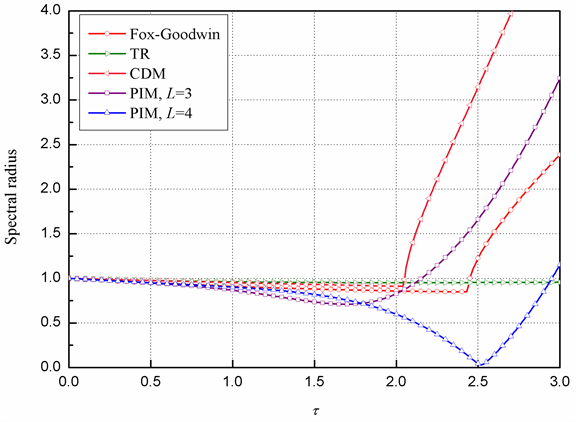Figure 2. Spectral radius ( $\xi$ = 0.1)

$\begin{array}{l}{\lambda }_{1,2}=A±B\text{i}={\text{e}}^{\left(-\stackrel{¯}{\xi }\stackrel{¯}{\omega }±\text{i}{\stackrel{¯}{\omega }}_{\text{d}}\right)h}\\ {\stackrel{¯}{\omega }}_{d}=\frac{\mathrm{arctan}\left(B/A\right)}{h}\\ \stackrel{¯}{\omega }=\frac{{\stackrel{¯}{\omega }}_{\text{d}}}{\sqrt{1-{\stackrel{¯}{\xi }}^{2}}}\\ \stackrel{¯}{\xi }=-\frac{\mathrm{ln}\left({A}^{2}+{B}^{2}\right)}{2\stackrel{¯}{\omega }h}\end{array}$ (28)

$\eta =\frac{\stackrel{¯}{T}-T}{T},\stackrel{¯}{T}=\frac{2\text{π}}{{\stackrel{¯}{\omega }}_{\text{d}}},T=\frac{2\text{π}}{{\omega }_{\text{d}}}$ (29)

4. 数值仿真

$\left[\begin{array}{ccc}1& 0& 0\\ 0& 1& 0\\ 0& 0& 1\end{array}\right]\left[\begin{array}{c}{\stackrel{¨}{x}}_{1}\\ {\stackrel{¨}{x}}_{2}\\ {\stackrel{¨}{x}}_{3}\end{array}\right]+\left[\begin{array}{ccc}2& -1& 0\\ -1& 2& -1\\ 0& -1& 2\end{array}\right]\left[\begin{array}{c}{x}_{1}\\ {x}_{2}\\ {x}_{3}\end{array}\right]=\left[\begin{array}{c}10\mathrm{sin}5t\\ 0\\ 0\end{array}\right]$ (30)

${x}_{\text{4}}=\text{sin5}t$ ，则方程(28)可转化为齐次方程

$\left[\begin{array}{cccc}1& 0& 0& 0\\ 0& 1& 0& 0\\ 0& 0& 1& 0\\ 0& 0& 0& 1\end{array}\right]\left[\begin{array}{c}{\stackrel{¨}{x}}_{1}\\ {\stackrel{¨}{x}}_{2}\\ {\stackrel{¨}{x}}_{3}\\ {\stackrel{¨}{x}}_{4}\end{array}\right]+\left[\begin{array}{cccc}2& -1& 0& -10\\ -1& 2& -1& 0\\ 0& -1& 2& 0\\ 0& 0& 0& 25\end{array}\right]\left[\begin{array}{c}{x}_{1}\\ {x}_{2}\\ {x}_{3}\\ {x}_{4}\end{array}\right]=\left[\begin{array}{c}0\\ 0\\ 0\\ 0\end{array}\right]$ (31)

${x}_{0}={\left[\begin{array}{cccc}0& 0& 0& 0\end{array}\right]}^{\text{T}},{\stackrel{˙}{x}}_{0}={\left[\begin{array}{cccc}0& 0& 0& 5\end{array}\right]}^{\text{T}}$ (32)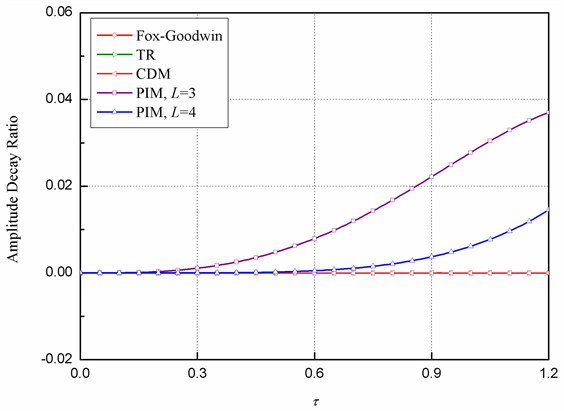Figure 3. Amplitude decay ratio ( ξ = 0)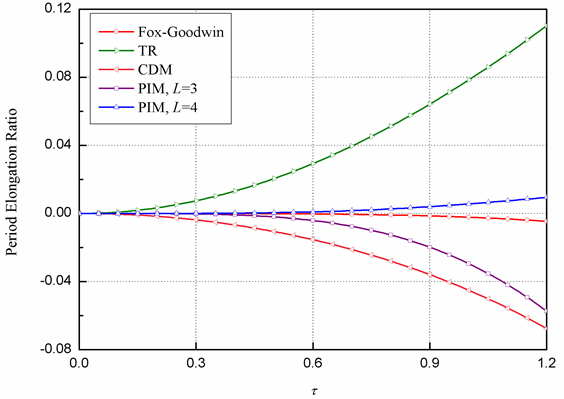Figure 4. Period elongation ratio ( ξ = 0)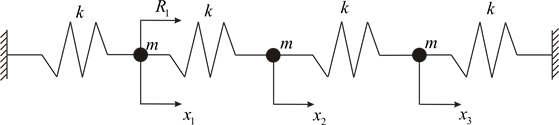Figure 5. Mass-spring system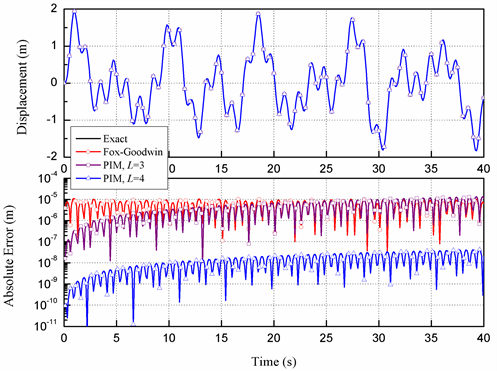Figure 6. Displacement and the absolute errors of the methods at node 1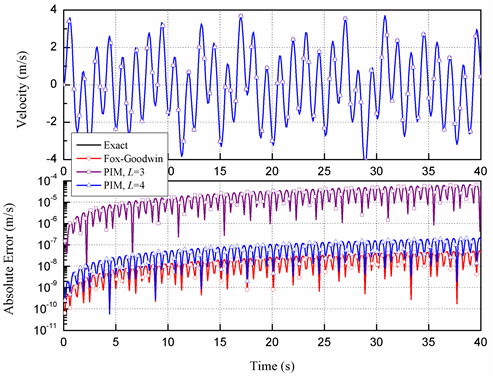Figure 7. Velocity and the absolute errors of the methods at node 1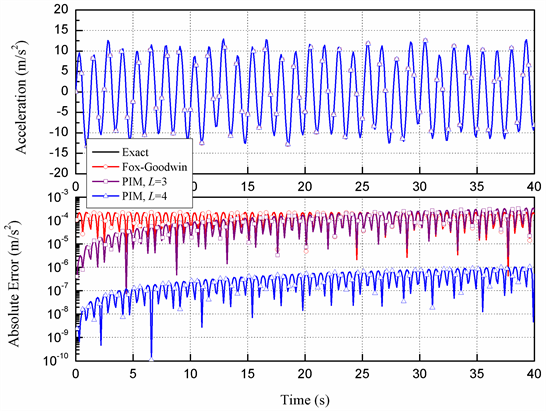Figure 8. Acceleration and the absolute errors of the methods at node 1

5. 结论

 Houbolt, J.C. (1950) A Recurrence Matrix Solution for the Dynamic Response of Elastic Aircraft. Journal of Aeronautical Science, 17, 540-550.
https://doi.org/10.2514/8.1722

 Newmark, N.M. (1959) A Method of Computation for Structural Dynamics. ASCE Journal of the Engineering Mechanics Divisions, 85, 67-94.

 Wilson, E.L. (1968) A Computer Program for Dynamic Stress Analysis of Underground Structures. SESM, University of California, Berkeley.

 Hilber, H.M., Hughes, T.J.R. and Taylor, R.L. (1977) Improved Numerical Dissipation for Time Integration Algorithms in Structural Dynamics. Earthquake Engineering and Structural Dynamics, 5, 283-292.
https://doi.org/10.1002/eqe.4290050306

 Wood, W.L., Bossak, M. and Zienkiewicz, O.C. (1980) An Alpha Modification of Newmark’s Method. International Journal for Numerical Methods in Engineering, 15, 1562-1566.
https://doi.org/10.1002/nme.1620151011

 Zhai, W.M. (1996) Two Simple Fast Integration Methods for Large-Scale Dynamic Problems in Engineering. International Journal for Numerical Methods in Engineering, 39, 4199-4214.
https://doi.org/10.1002/(SICI)1097-0207(19961230)39:24<4199::AID-NME39>3.0.CO;2-Y

 Chung, L. and Hulbert, G.M. (1993) A Time Integration Algorithm for Structural Dynamics with Improved Numerical Dissipation: The Generalized-α Method. Journal of Applied Mechanics, 60, 371-375.
https://doi.org/10.1115/1.2900803

 Bathe, K.J. and Baig, M.M.I. (2005) On a Composite Implicit Time Integration Procedure for Nonlinear Dynamics. Computers and Structures, 83, 2513-2524.
https://doi.org/10.1016/j.compstruc.2005.08.001

 Zhou, X. and Tamma, K.K. (2004) Design, Analysis, and Synthesis of Generalized Single Step Single Solve and Optimal Algorithms for Structural Dynamics. International Journal for Numerical Methods in Engineering, 59, 597-668.
https://doi.org/10.1002/nme.873

 钟万勰. 结构动力方程的精细时程积分[J]. 大连理工大学学报, 1994, 34(2): 131-136.

 钟万勰. 暂态历程的精细计算方法[J]. 计算结构力学及其应用, 1995, 12(1): 1-6.

 Lin, J.H., Shen, W.P. and Williams, F.M. (1997) Accurate High-Speed Computation of Nonstationary Random Seismic Response. Engineering Structures, 19, 586-593.
https://doi.org/10.1016/S0141-0296(97)83154-9

 汪梦甫, 周锡元. 结构动力方程的更新精细积分方法[J]. 力学学报, 2004, 36(2): 191-195.

 顾元宪, 陈飚松, 张洪武. 结构动力方程的增维精细积分法[J]. 力学学报, 2000, 32(4): 447-456.

 Wang, M.F. and Au, F.T.K. (2006) Assessment and Improvement of Precise Time Step Integration Method. Computers and Structures, 84, 779-786.
https://doi.org/10.1016/j.compstruc.2006.02.001

 Fox, L. and Goodwin, E.T. (1949) Some New Methods for the Numerical Integration of Ordinary Differential Equations. Proceedings of Cambridge Philosophical Society, 45, 373-388.
https://doi.org/10.1017/S0305004100025007

Top